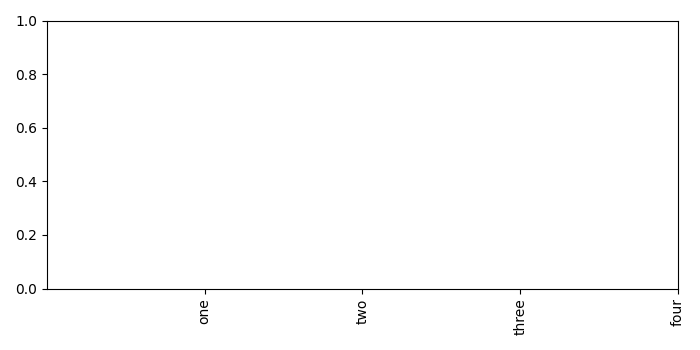# How can I rotate xtick labels through 90 degrees in Matplotlib?

To rotate xtick labels through 90 degrees, we can take the following steps −

• Make a list (x) of numbers.

• Add a subplot to the current figure.

• Set ticks on X-axis.

• Set xtick labels and use rotate=90 as the arguments in the method.

• To display the figure, use show() method.

## Example

import matplotlib.pyplot as plt
plt.rcParams["figure.figsize"] = [7.00, 3.50]
plt.rcParams["figure.autolayout"] = True
x = [1, 2, 3, 4]
ax1 = plt.subplot()
ax1.set_xticks(x)
ax1.set_xticklabels(["one", "two", "three", "four"], rotation=90)
plt.show()

## Output